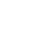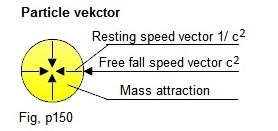Frontpage Search Email . Danish .Welcome to my homepage, developing the classic head physicsMass formation If you have not put yourself into the system that I use, then understanding the basis for this section is not present. This section is a continuation of the classic Big Bang section.The shift in the thermal balance of the universe causes a span which has two velocity vectors, free fall c and resting span 1 / c (see classic big bang fig. b.36).The vectors have the same value, measured in energy, but must be kept separate in the mathematical system because the volume from which the energy is calculated does not have the same state. Normally: free fall speed vector m = Vx * c2, the volume is taken out from the thermal resting point of the universe.Mass: dormant speed vector m = Vx * (1/c)2, the volume is taken from the compressed volume. ..................................If the particle mass is not at rest and is affected by a force, then the vectors and volume must be corrected.With the speed of light, the universe can gather millions of parts to the absolute accuracy. We use a unit-controlled number system, we cannot assemble two parts to the absolute accuracy even though we spend unlimited time on it. This means that it is not possible to assemble the parts of the universe mathematically without also using combination solutions, but it does not change that we need to know how the individual parts are put together.The core of the universe:The universe can only create one particle in the Big Bang process (see classic big bang figure 3.1).I call the particle a basic core. The mass of the basic core is calculated as follows: .............mbasis = ((4/3) * pi *  r3) *  c2 = 5.185681022 * 10-34kg.Many years ago I made a very thorough study of the nucleus of the atom. Here it was found that the energy of the nucleus is stabilized by the binding structure of the nucleons (see Atomic binding Fig. 1.8).The binding structure is the way of the universe to adapt the nuclei to the surrounding space and can be found in almost all structures, including in molecular crystals. ...........................................A proton consists of approx. 3 million basic cores.When a proton gets crushed in a particle accelerator, the weakest joints will collapse first, making all the fragments not fall apart at the same time, but will divide into smaller and smaller pieces. The images coming from the accelerator are completely in line with the system used here. The problem is how to handle it mathematically.Coordinators and Vectors of the Universe:All function vectors in the Universe always refer to the resting point of the universe.It is therefore appropriate to use a coordinate system which indicates the speed vector reference to the 0 point. The volume of the universe is therefore allocated an infinite number of points, the coordinate will look like this next to the 0 point of the universe (0, 0, 0,) ........................................You will typically encounter the problem of emission of radiation in particle velocities and, incidentally, the universe is a huge, cohesive network of vectors that all refer to the 0 point of the universe.When a particle lies still and is not affected by any forces, it will have the shape of a ball.The velocity vector of the particle points to the center, which makes it stand upside down in relation to the normal function of the Universe.FIG. p329 shows two velocity vectors having different length, free fall c and compressed 1 / c. When a particle is applied kinetic energy, the compressed portion will be displaced and free fall vector correspondingly reduced, resulting in the particle changing shape. You should be aware that mass attraction is a speed variable. ......................Here I have shown the basic part in partial speed. Underlying is that a particle is made up of basic cores  and here we have the particle's deceleration and the emission function of the radiation.I just want to show what is to be integrated into the system:There are a number of functions associated with moving particles. Example: ........................You can read more about this in my section about Particle Radiation. ...............................We can now see the underlying function parts of the universe. There is not taken position  here about how you can put them into your projects, but you can see more about the combined composition in my other sections. Last updated  march 01, 2019 Email : info@jwhdk.euClassic big bang. Mass formation. Atom structure. Atom binding. Molecular binding. Particle radiation Magnetic fields. Mass attraction. Conclusion. Micro physics